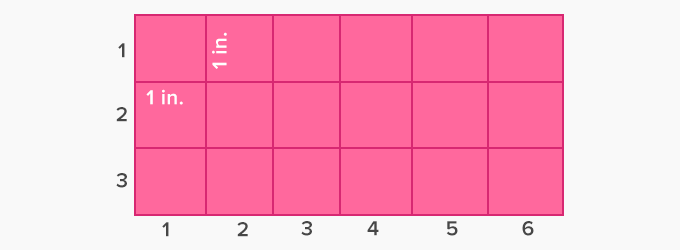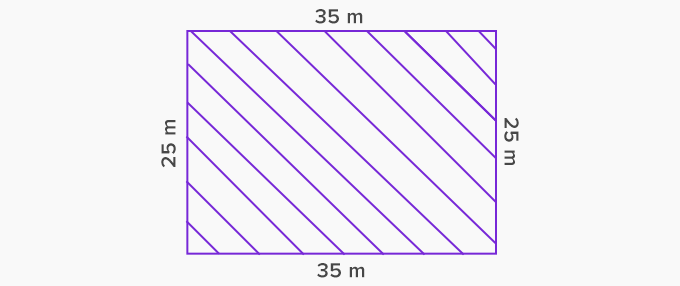Area Of A Rectangle - Definition with Examples

The Complete K-5 Math Learning Program Built for Your Child

• 30 Million Kids

Loved by kids and parent worldwide

• 50,000 Schools

Trusted by teachers across schools

• Comprehensive Curriculum

Aligned to Common Core

What is Area of a Rectangle?

The area of a two-dimensional shape is the space occupied by the shape. In the given rectangle, the shaded space is the area of the rectangle.Finding the area of a rectangular wall helps us in finding the amount of color required to paint it. Likewise, we can calculate the space occupied by a rectangular lawn in a park by finding the area of the rectangle. We measure the area in square units.

By finding the number of unit squares that are required to fill a shape, we can calculate its area. Since each unit square occupies one square unit space, so the total number of unit squares in the shape gives its area.

Consider a rectangle of length 6 in. and width 3 in. It can be filled with 3 rows and 6 columns of unit squares.The rectangle can be shown on top of a 10x10 grid with shaded inside the rectangle.

Each of these squares has an area of 1 square inch, and in the rectangle, there are 18 such squares. So, the area of the rectangle is 18 square inches. In other words, the area of a rectangle is the product of its length and width. That is, A = l x w where l is the length and w is the width of the rectangle.

For example, the area of a rectangle of length 35 m and width 25 m is 35 times 25 or 875 square meters.A rectangular room has a length of 16 feet and a width of 18 feet. How much carpet is required to cover the entire room?The area of the carpet is equal to the area of the room. We can find the area of the room by multiplying its length by width. That is, 16 ft times 18 ft or 288 square feet. Therefore, to cover the room, 288 square feet of carpet is required.

 Fun Facts The square is a special rectangle where length and width are the same. Hence the area of a square is given by multiplying the length of each side by itself.

Won Numerous Awards & Honors